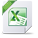# Pianotech

View Only

## Pure 12th Equal Temperament

• #### 1.  Pure 12th Equal Temperament

Posted 05-09-2019 23:04

### Pure 12th Equal Temperament

 Note Cents C 0.00 C# 100.10 D 200.21 D# 300.31 E 400.41 F 500.51 F# 600.62 G 700.72 G# 800.82 A 900.93 A# 1001.03 B 1101.13 C 1201.23 C# 1301.34 D 1401.44 D# 1501.54 E 1601.65 F 1701.75 F# 1801.85 G 1901.96

The exact size in cents of any interval of Pure 12th Equal Temperament can be calculated by using the following formula:

1200 × log23 × N/19

N is the number of Semitones within an interval.

For example, N = 7 for all Fifths because each Fifth contains 7 Semitones, N = 12 for all Octaves because each Octave contains 12 Semitones and N = 19 for all Twelfths because each Twelfth contains 19 Semitones:

Semitone = 1200 × log23 × 1/19 = 100.10 cents.

Fifth = 1200 × log23 × 7/19 = 700.72 cents.

Octave = 1200 × log23 × 12/19 = 1201.23 cents.

Twelfth = 1200 × log23 × 19/19 = 1901.96 cents.

------------------------------
Roshan Kakiya
------------------------------

Attachment(s)